# Earth Geometry

Earth geometry is a mathematical concept that deals with the spherical Earth in relation to distance, time and location of places which are found on the latitude or longitudes

## Earth Geometry Fundamentals

The Earth is made up of two demarcation of line namely the latitude and the longitude

## what you should know about the latitude

latitude: are lines which run from east to west of the earth

The latitude which is 0 degrees is refered to has the equator

The signs for the line on the latitudes are N (north) or S (south)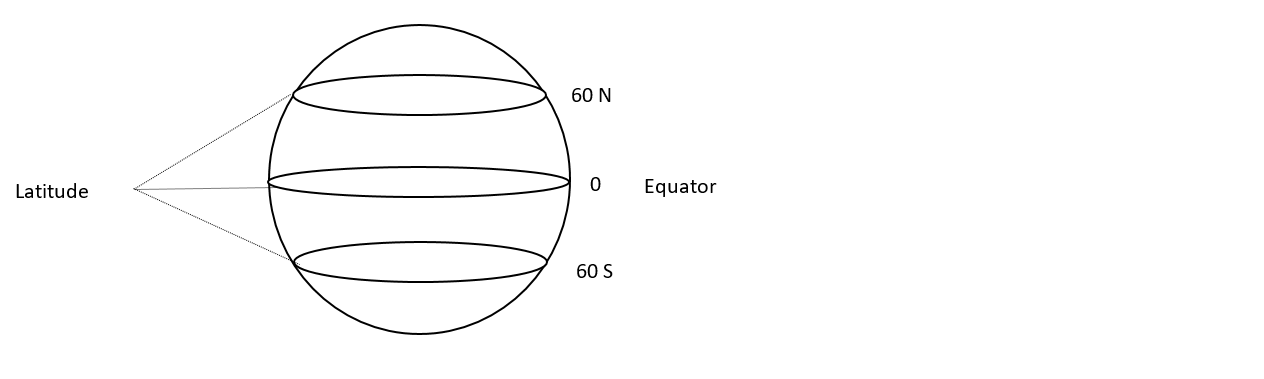## what you should know about the longitude

longitude: are lines which run from north to south of the earth

The longitude which is 0 degrees is refered to has the Greenwich meridian

The signs for the line on the longitude are E (east) or W (west)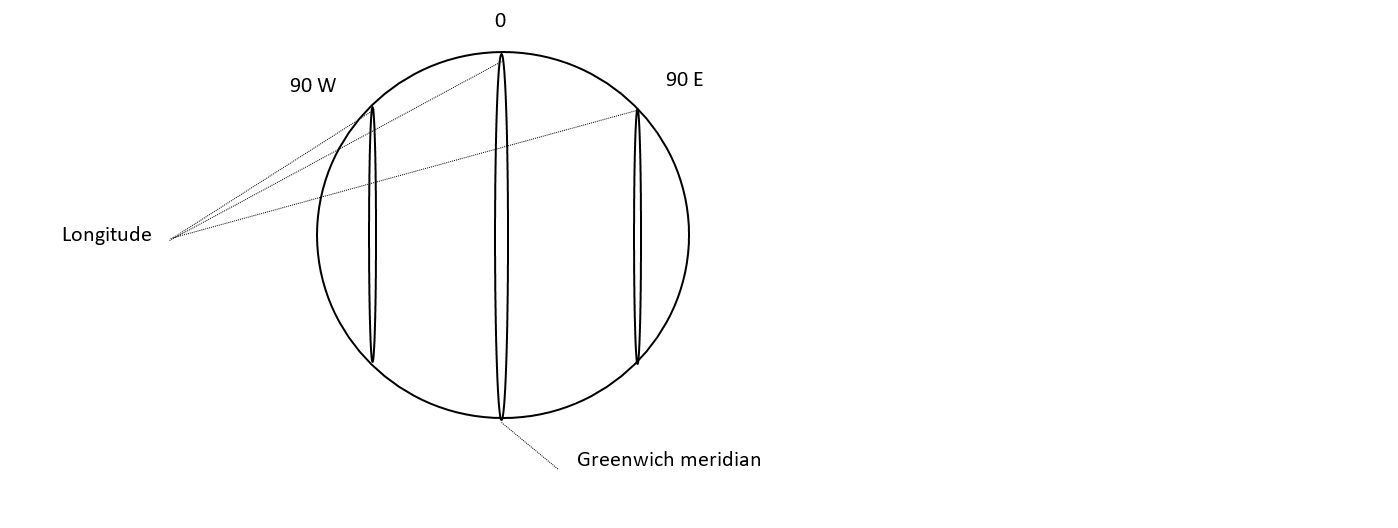## How to Calculate Time in earth Geometry

1. First step to take to calculate the time between two difference places is to find the difference between those two places which can be the longitudes or latitudes

2. when finding the difference between two places you have to know when to add or subtract two points:

if the locations are found in the same region you have to subtract those two places example of places which are: ${\mathrm{50}}^{o}$ E and ${\mathrm{80}}^{o}$E and given that the time at ${\mathrm{50}}^{o}$ E is 12:00 hrs what is the time at ${\mathrm{80}}^{o}$ E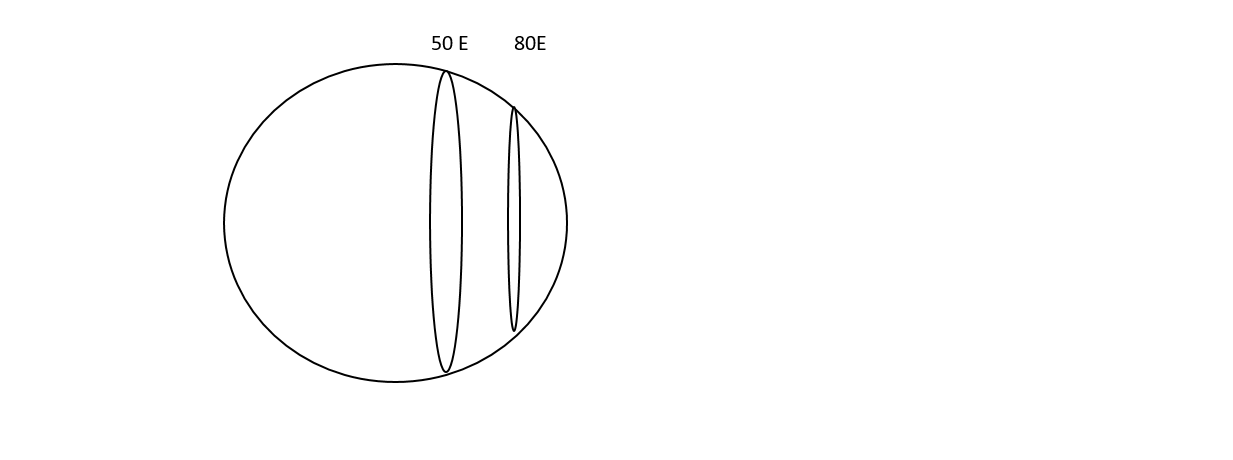The difference between the two places:

difference = ${\mathrm{80}}^{o}$ - ${\mathrm{50}}^{o}$

difference = ${\mathrm{30}}^{o}$

hours = $\frac{{\mathrm{30}}^{o}}{{\mathrm{15}}^{o}}$

hours = $2 hours$

Therefore time at ${\mathrm{80}}^{o}$ E is 12:00 + 2:00 = 14:00 hrs

if the places are in difference locations the difference is found by adding the values of those two places example are:${\mathrm{20}}^{o}$ W and ${\mathrm{120}}^{o}$E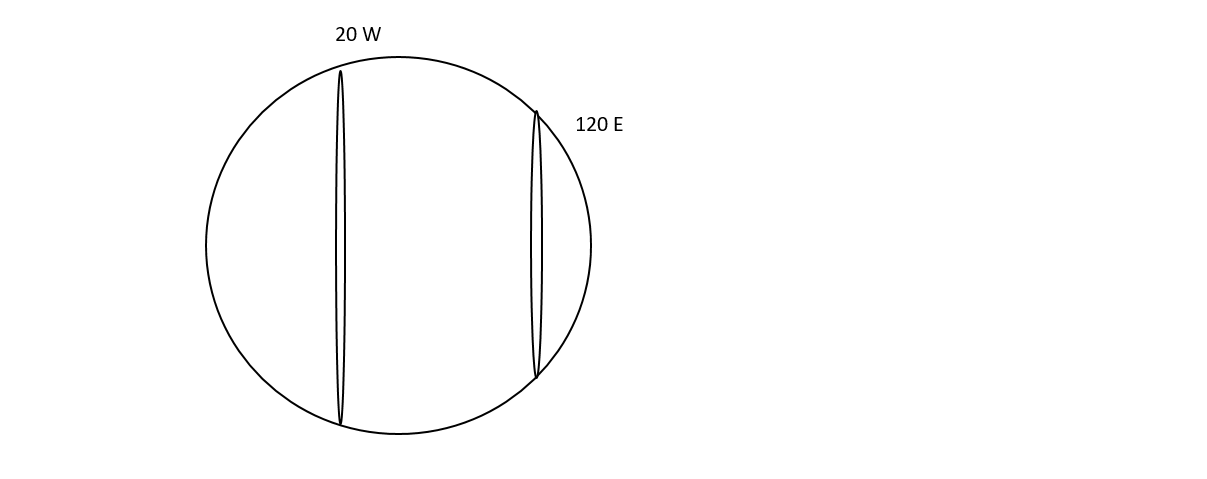Given that the time at ${\mathrm{120}}^{o}$ E is 18:00 hrs what is the time at ${\mathrm{20}}^{o}$ W

difference = ${\mathrm{120}}^{o}$ + ${\mathrm{20}}^{o}$

difference = ${\mathrm{140}}^{o}$

hours = $\frac{{\mathrm{140}}^{o}}{{\mathrm{15}}^{o}}$ = 9.33

= $9 hours$ and a remainder of ${5}^{o}$

${1}^{o}$ = 4 minutes

${5}^{o}$ = x minutes

x = 9 minutes

09:09 hrs

The time of the place which is in the west having ${\mathrm{20}}^{o}$ W is 18:00 - 09:09 hrs = 08:51 hrs

## Points to note

1. To find the time of a place which is in the east, given that you have been provided with the time of a place in the west. The time difference is added to the time of a place in the west to find time of a place in east

2. Secondly to find the time of a place which is in the west, provided that you have been given the time of a place in the east. The time difference is subtracted to the time of a place in the east to find time of a place in west

3. ${\mathrm{15}}^{o}$ is equal to 1 hour

4. Fourthly is to divide ${\mathrm{15}}^{o}$ into the difference find between the latitude or the longitudes

5. if they are any remainders when dividing with ${\mathrm{15}}^{o}$ take note that the remainders are minutes.

6. ${1}^{o}$ is equal to 4 minutes therefore multiply the remainder with 4 to find the minutes

## Example 1

In the diagram, find: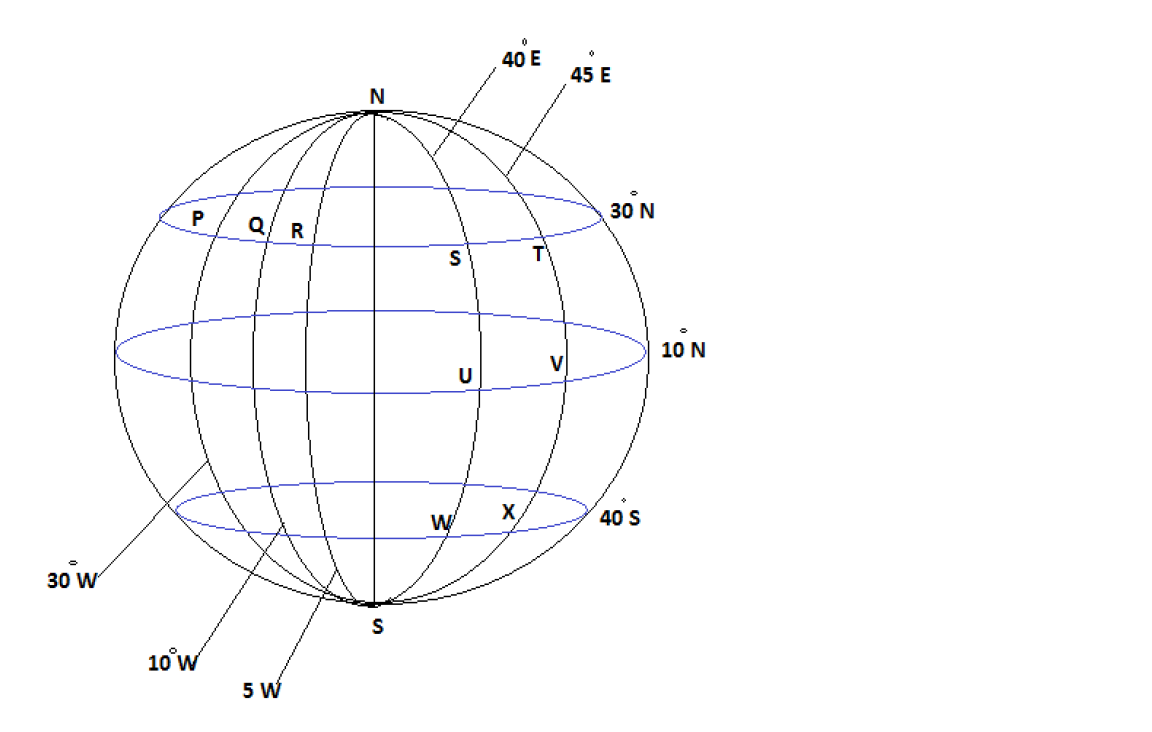(i) Describe position Q, W and V

(ii) The difference in longitude between P and Q ; P and T

(iii) The difference in latitude between S and U; S and W

Solutions

(i)

Q = ${\mathrm{30}}^{o}$N, ${\mathrm{10}}^{o}$W

W = ${\mathrm{40}}^{o}$S, ${\mathrm{40}}^{o}$E

V = ${\mathrm{10}}^{o}$S, ${\mathrm{45}}^{o}$E

(ii)

P and Q

P = ${\mathrm{30}}^{o}$W, Q = ${\mathrm{10}}^{o}$W

P and Q = ${\mathrm{30}}^{o}$W - ${\mathrm{10}}^{o}$W

P and Q = ${\mathrm{20}}^{o}$

p and T

p = ${\mathrm{30}}^{o}$W, T = ${\mathrm{45}}^{o}$E

p and T = ${\mathrm{30}}^{o}$W, + ${\mathrm{45}}^{o}$E

p and T = ${\mathrm{75}}^{o}$

(iii)

S and U

S = ${\mathrm{30}}^{o}$N, U = ${\mathrm{10}}^{o}$N

S and U = ${\mathrm{30}}^{o}$N - ${\mathrm{10}}^{o}$N

S and U = ${\mathrm{20}}^{o}$

S and W

S = ${\mathrm{30}}^{o}$N, W = ${\mathrm{40}}^{o}$S

S and W = ${\mathrm{30}}^{o}$N + ${\mathrm{40}}^{o}$S

S and W = ${\mathrm{70}}^{o}$

## Example 2

W, X, Y and Z are four points on the surface of the earth as shown in the diagram below.

[𝜋 = 3.142,𝑅 = 3437𝑛𝑚]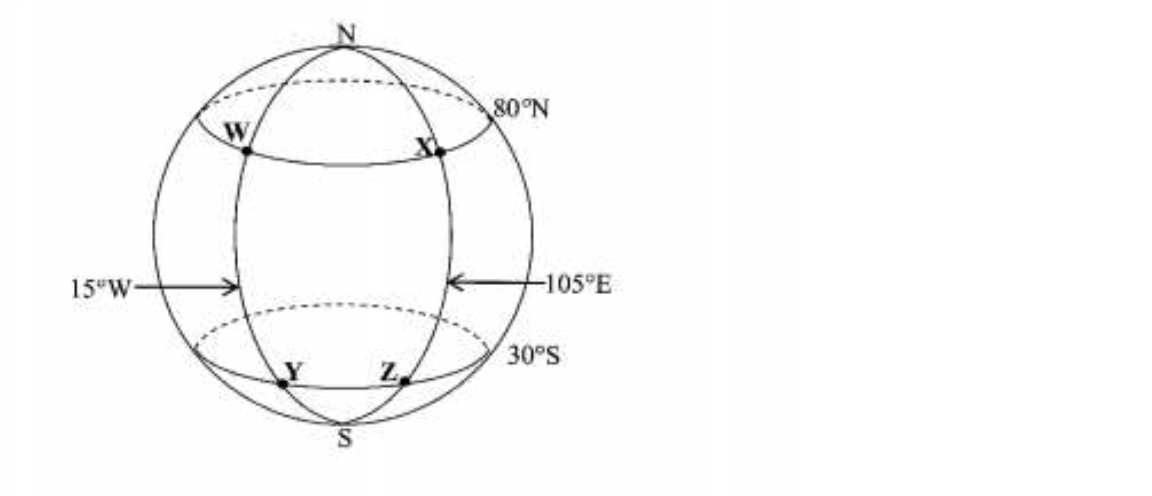(i) Calculate the difference in latitude betweeen W and Y.

(ii) Calculate the distance in nautical miles between

(a) X and Z along the longitude 105⁰E

(b) Y and Z along the circle of latitude 30⁰S

Solution:

(i)

W = ${\mathrm{80}}^{o}$N, ${\mathrm{30}}^{o}$S

W = ${\mathrm{80}}^{o}$N, + ${\mathrm{30}}^{o}$S

Answer: ${\mathrm{110}}^{o}$

(ii)

##### How to find distance in earth geometry

(a)

Distance XZ

Formula

$\mathrm{D =}\frac{\theta }{360}×2\pi R$

$\pi$ = 3.142, R = 3437nm, $\theta$ = 80⁰N + 30⁰S = 110,

$\mathrm{XZ =}\frac{\mathrm{110}}{360}×2×3.142×\mathrm{3437}$

$\mathrm{XZ =}\frac{\mathrm{2375791.88}}{360}$

$\mathrm{XZ =}6599.42$

Answer: $\mathrm{XZ =}6600nm$

(b)

Distance YZ

Formula

$\mathrm{D =}\frac{\theta }{360}×2\pi r{\mathrm{CosX}}^{o}$

##### Note that

R = r ${\mathrm{Cos\left(x\right)}}^{o}$ meaning that capital R (Radius) is equal to small r times cos(x)⁰. This is applied when finding distance betweeen latitudes

$\pi$ = 3.142, r = 3437nm, $\theta$ = 15⁰W + 105⁰E = 120, ${\mathrm{Cos\left(x\right)}}^{o}$ = ${\mathrm{Cos\left(30\right)}}^{o}$

$\mathrm{YZ =}\frac{\mathrm{120}}{360}×2×3.142×\mathrm{3437}×{\mathrm{Cos\left(30\right)}}^{o}$

$\mathrm{YZ =}\frac{\mathrm{2244541.224}}{360}$

$\mathrm{YZ =}6234.836$

Answer: $\mathrm{YZ =}6235nm$

## Points to note

$\theta$ = theta represents the difference in latitude or longitude

$\pi$ = Pi represents $\frac{\mathrm{22}}{7}$ or 3.142

$\mathrm{Distance =}\frac{\theta }{360}×2\pi R$ = Formula to find the distance between longitudes

$\mathrm{Distance =}\frac{\theta }{360}×2\pi r{\mathrm{CosX}}^{o}$ = Formula to find the distance between latitudes

R = r ${\mathrm{Cos\left(x\right)}}^{o}$

## How to solve earth geometry

The diagram below shows three points P, Q and R on the surface of the earth.

[Take $\pi$ as 3.142 and R = 6370km ]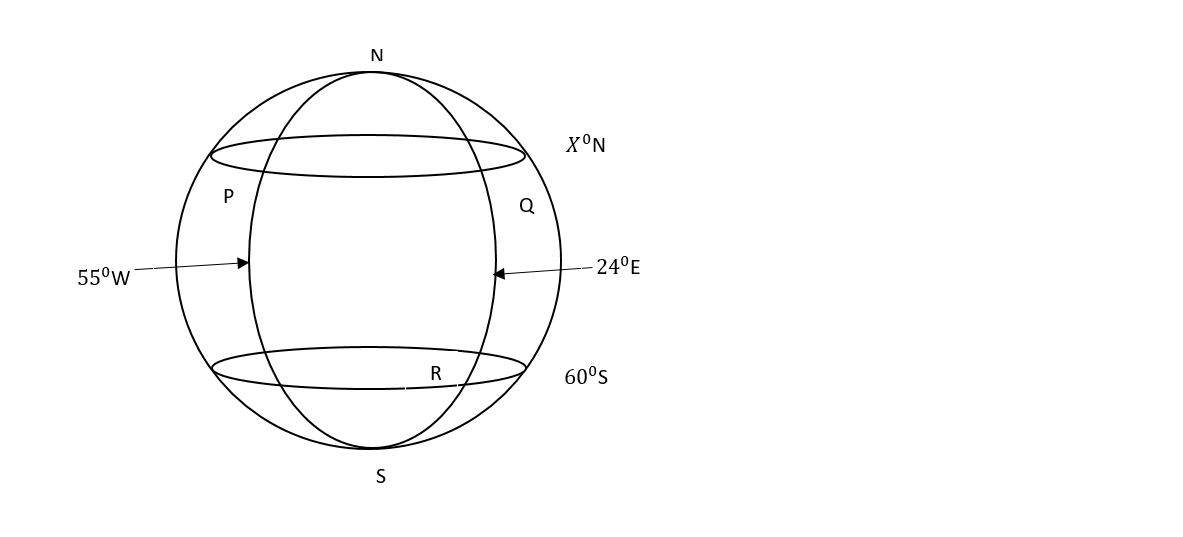(a) Calculate the difference in longitude between P and R

(b) Given that the distance between P and Q on latitude ${X}^{o}N$ is 4392.079611km, calculate X.

(c) Calculate the distance QR on longitude ${\mathrm{24}}^{o}E$.

Solution:

(a)

P = 55 W

R = 24 E

difference = $\mathrm{55}+24$

Answer: ${\mathrm{79}}^{o}$

(b)

Formula

$\mathrm{D =}\frac{\theta }{360}×2\pi R{\mathrm{CosX}}^{o}$

D = 4392.079611

$\theta$ = 79

$\pi$ = 3.142

R = 6370

${\mathrm{CosX}}^{o}$ = ?

$\mathrm{D =}\frac{\theta }{360}×2\pi R{\mathrm{CosX}}^{o}$

$\mathrm{4392.08 =}\frac{\mathrm{79}}{360}×2×3.142×\mathrm{6370}{\mathrm{CosX}}^{o}$

$\mathrm{4392.08 =}8784.159{\mathrm{CosX}}^{o}$

${\mathrm{CosX}}^{o}=\mathrm{0.5}$

${X}^{o}={\mathrm{Cos}}^{-1}\mathrm{0.5}$

Answer: $X={\mathrm{60}}^{o}N$

(c)

Formula

$\mathrm{D =}\frac{\theta }{360}×2\pi R$

QR = ?

Q = 60

R = 60

$\theta$ = 120

$\pi$ = 3.142

$\mathrm{QR =}\frac{\mathrm{120}}{360}×2×3.142×\mathrm{6370}$